Courses

# Bending And Shear Stress - 2

## 8 Questions MCQ Test Mock Test Series for Civil Engineering (CE) GATE 2020 | Bending And Shear Stress - 2

Description
This mock test of Bending And Shear Stress - 2 for Civil Engineering (CE) helps you for every Civil Engineering (CE) entrance exam. This contains 8 Multiple Choice Questions for Civil Engineering (CE) Bending And Shear Stress - 2 (mcq) to study with solutions a complete question bank. The solved questions answers in this Bending And Shear Stress - 2 quiz give you a good mix of easy questions and tough questions. Civil Engineering (CE) students definitely take this Bending And Shear Stress - 2 exercise for a better result in the exam. You can find other Bending And Shear Stress - 2 extra questions, long questions & short questions for Civil Engineering (CE) on EduRev as well by searching above.
QUESTION: 1

Solution:
QUESTION: 2

### A beam of square cross -section ( S x B) is used as a beam with one diagonal horizontal. The location of the maximum shear stress from the neutral axis will be at distance o

Solution:

The digonal is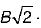The location of maximum shear stress from neutral axis is at a distance of 1/8   length of diagonal i.e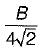QUESTION: 3

### Which one of the following diagrams indicates the shear stress distribution for the beam as shown in the figure below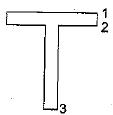Solution:
QUESTION: 4

A beam is of i-section with flanges 200 mm x 10 mm and web 180 mm x 10 mm. Due to the bending moment applied on the beam section, maximum stress developed in the beam section is 100 MPa, what is the stress developed at inner edge of the flange?

Solution: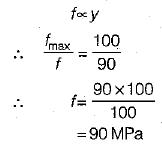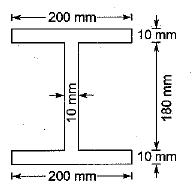QUESTION: 5

A MS beam is subjected to a bending moment, such that a stress of 100 MPa is developed in a layer at a distance of 100 mm from the neutral layer. If E= 200 GPa, what is the radius of curvature of the beam?: .

Solution: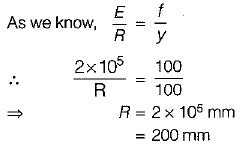QUESTION: 6

A cantilever of uniform strength σ, having rectangular section of constant breadth b but variable depth d is subjected to a UDL throughout its length. If the depth of the section is 150 mm at the fixed end, then what is the depth of the middle of the length of cantilever

Solution:
QUESTION: 7

A beam with a square section of 80 mm x 80 mm is simply supported at its ends. A load W is applied at the centre of the beam. If the maximum shear stress developed in the beam section is 6 N/mm2. What is the magnitude of W?

Solution:
QUESTION: 8

A l-section is subjected to transverse shear force. At which layer maximum shear stress is developed?

Solution: